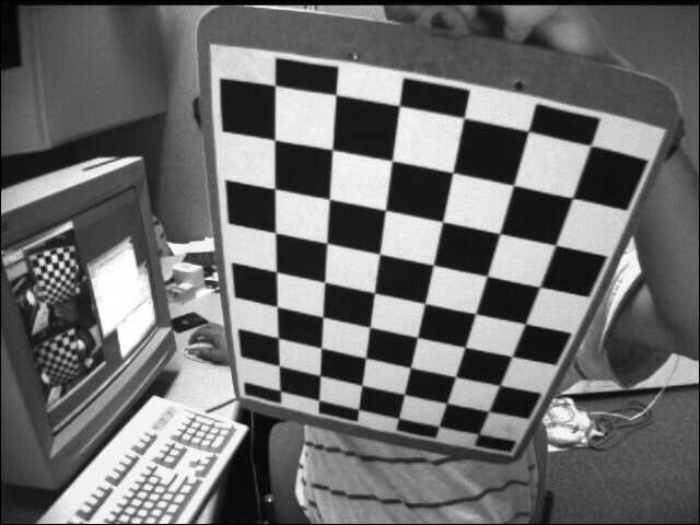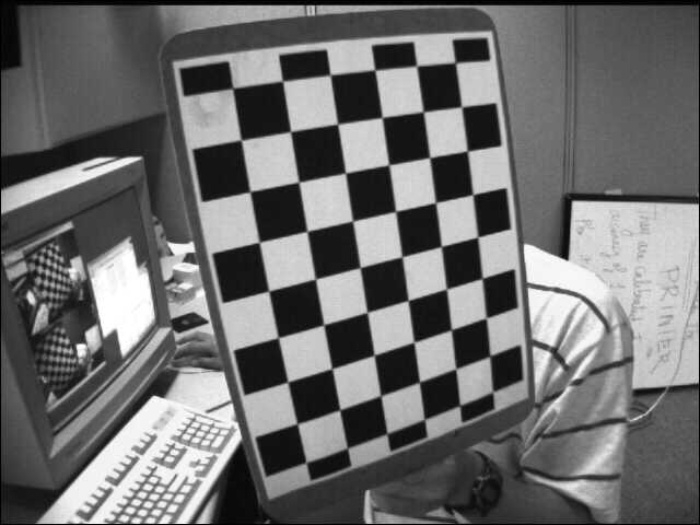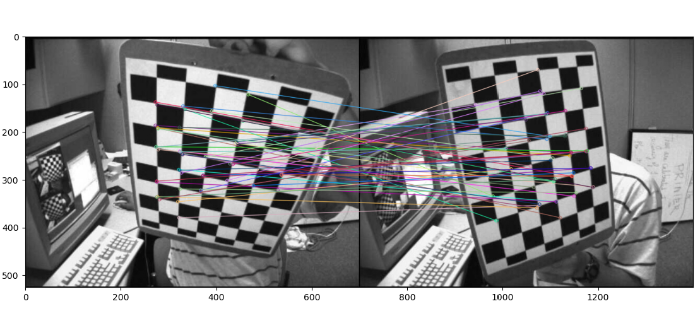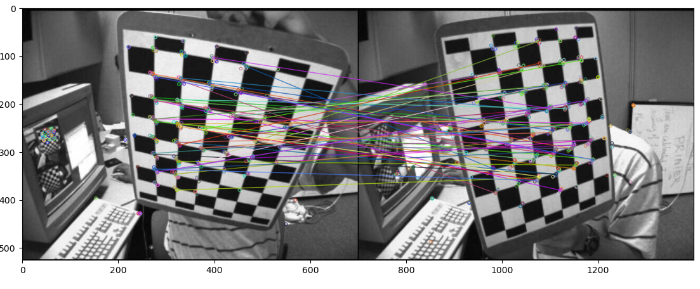# OpenCV Python – Matching the key points of two images using ORB and BFmatcher

To match the keypoints of two images, we use ORB (Oriented FAST and Rotated BRIEF) to detect and compute the feature keypoints and descriptors and Brute Force matcher to match the descriptors in both images.

## Steps

To match keypoints of two images using the ORB feature detector and Brute Force matcher, you could follow the steps given below −

• Import the required libraries OpenCV, Matplotlib and NumPy. Make sure you have already installed them.

• Read two input images using cv2.imread() method as grayscale images. Specify the full path of the image.

• Initiate ORB object orb with default values using orb=cv2.ORB_create().

• Detect and compute the keypoints 'kp1' & 'kp2' and descriptors 'des1' & 'des2' in the both input images using orb.detectAndCompute().

• Create a BFmatcher object as bf = cv2.BFMatcher() and match the descriptors using this BFmatcher object as bf.match(des1, des2). It returns the matches. Sort the matches in order of their distances.

• Draw the matches on the original input images using cv2.drawMatches().

• Visualize the keypoint matches.

Let's look at some examples to match keypoints of two images using the ORB feature detector and Brute Force matcher.

## Input Images

We use the following images as input files in the examples below.## Example

In this example, we detect the keypoints and descriptors of the two input images using ORB algorithm and match the descriptors using the Brute Force matcher. Also we draw the best 50 keypoint matches. In this example we pass flags=2 to drawMatches() to draw matches.

# import required libraries
import numpy as np
import cv2
from matplotlib import pyplot as plt

# read two input images as grayscale

# Initiate ORB detector
orb = cv2.ORB_create()

# detect and compute the keypoints and descriptors with ORB
kp1, des1 = orb.detectAndCompute(img1,None)
kp2, des2 = orb.detectAndCompute(img2,None)

# create BFMatcher object
bf = cv2.BFMatcher(cv2.NORM_HAMMING, crossCheck=True)

# Match descriptors.
matches = bf.match(des1,des2)

# print(matches)

# Sort them in the order of their distance.
matches = sorted(matches, key = lambda x:x.distance)

# Draw first 50 matches.
img3 = cv2.drawMatches(img1,kp1,img2,kp2,matches[:50], None, flags=2)
plt.imshow(img3),plt.show()


## Output

On execution, it will produce the following output## Example

In this example, we detect the keypoints and descriptors of the two input images using ORB algorithm and match the descriptors using the Brute Force matcher. Also we draw the best 50 keypoint matches. In this example we pass flags=0 to drawMatches() to draw matches.

# import required libraries
import numpy as np
import cv2
import matplotlib.pyplot as plt

# read two input images as grayscale

# Initiate ORB detector
orb = cv2.ORB_create()

# detect and compute the keypoints and descriptors with ORB
(kp1,des1) = orb.detectAndCompute(img1, None)
(kp2,des2) = orb.detectAndCompute(img2, None)

# create BFMatcher object
bf = cv2.BFMatcher(cv2.NORM_HAMMING, crossCheck=True)

# Match descriptors.
matches = bf.match(des1,des2)

# Sort them in the order of their distance. Least distance is better
matches = sorted(matches, key=lambda val: val.distance)

# Draw first 50 matches.
out = cv2.drawMatches(img1, kp1, img2, kp2, matches[:50], None, flags=0)
plt.imshow(out), plt.show()


## Output

On execution, it will produce the following outputNotice the differences between the keypoints drawn in output images in both the examples.

Updated on: 05-Dec-2022

2K+ Views